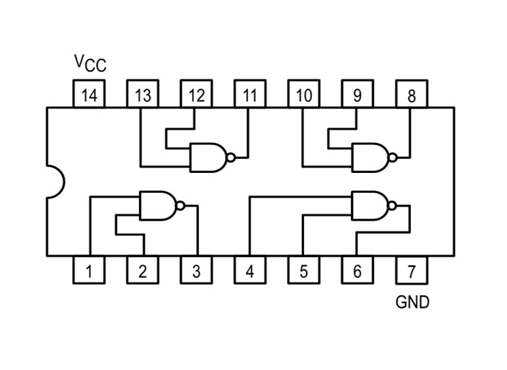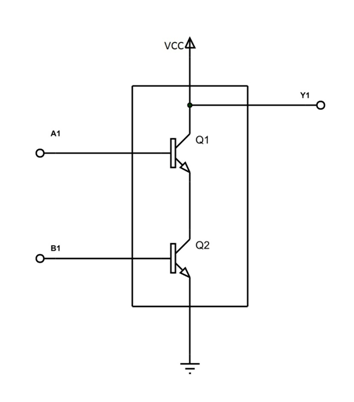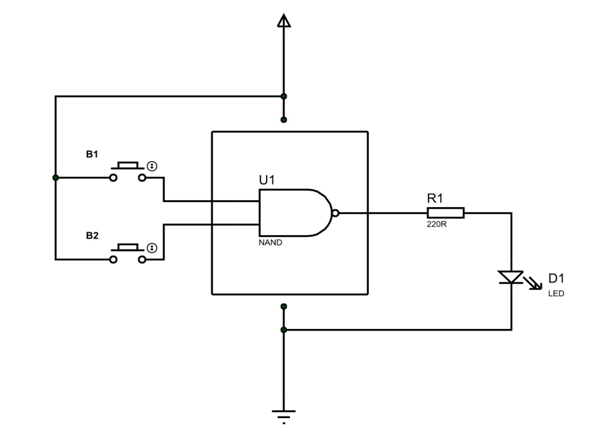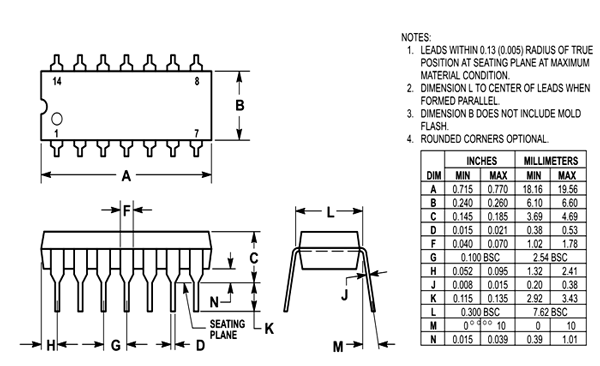# 74LS00–Quad Two Input NAND Gate

74LS00 is a member of 74XXYY IC series. The 74xxyy ICs are logic gates of digital electronics. 74LS00 IC has four NAND gates. Also each gate has two inputs. Hence name QUAD TWO INPUT NAND GATE.

### 74LS00 Pin Configuration

74LS00 is a 14 PIN device. The chip is available in different packages and is chosen depending on requirement. The description for each pin is given below.

 Pin Number Description NAND GATE 1 1 A1-INPUT1 of GATE 1 2 B1-INPUT2 of GATE 1 3 Y1-OUTPUT of GATE1 NAND GATE 2 4 A2-INPUT1 of GATE 2 5 B2-INPUT2 of GATE 2 6 Y2-OUTPUT of GATE2 NAND GATE 3 9 A3-INPUT1 of GATE 3 10 B3-INPUT2 of GATE 3 8 Y3-OUTPUT of GATE3 NAND GATE 4 12 A4-INPUT1 of GATE 4 13 B4-INPUT2 of GATE 4 11 Y4-OUTPUT of GATE4 SHARED TERMINALS 7 GND- Connected to ground 14 VCC-Connected to positive voltage to provide power to all four gates

### 74LS00 Features and Specifications

• Operatingvoltage range: +4.75 to +5.25V
• Maximum supply voltage:7V
• Maximum current allowed to draw through each gate output: 8mA
• TTL outputs
• Maximum ESD: 3.5KV
• Typical Rise Time: 15ns
• Typical Fall Time: 15ns
• Operating temperature:0°C  to 75°C

### 74LS00 Equivalents

SN54LS00, SN7400, CD4011, Any two transistors can be reconfigured to form a NAND gate.

### Where 74LS00 IC is Used?

There are many reasons of using 74LS00 in electronic circuits. Here are a few examples where it is used.

1. 74LS00 basically used for performing NAND function. The IC has four NAND gates in it. Each gate can be used separately.

2. When you want logic inverter. NAND gates in this chip can be reconfigured to make them NOT gate.  So we can make 74LS00 a four NOT gate chip if necessary.

3. Where high speed NAND operation is necessary. This chip has less transition times which are needed for high speed applications. So 74LS00 can be used in high frequency systems.

4. 74LS00 is one of the cheapest IC. It is really popular and is available everywhere.

### How to Use 74LS00 IC

As mentioned earlier 74LS00 has four NAND gates. The internal connection of four gates is shown below.Now it is good to remind you that NAND gate is combination of AND gate and NOT gate.

So NAND = AND + NOT.

The truth table of NAND gate is given as,

 Input1 Input2 AND Output NAND Output LOW LOW LOW HIGH HIGH LOW LOW HIGH LOW HIGH LOW HIGH HIGH HIGH HIGH LOW

For understanding the NAND gate responses let us study the internal circuitry of NAND gate.In the circuit when both inputs A1 and B1 are LOW:

Both transistors Q1 and Q2 will be OFF. So the total supply voltage appears across the transistors Q1 and Q2. Because output Y1 is nothing but voltage across transistors Q1 and Q2, Y1 will be HIGH.

In the case when any one input is HIGH:

Only the relative transistor will be ON leaving the other OFF. With that the entire supply voltage appears across the transistor which is in OFF state. Because output Y1 is voltage across both transistors Q1 and Q2, Y1 will be HIGH.

When both inputs are HIGH:

Both transistors will be ON and voltage across both of them will be zero.Because output Y1 is voltage across both transistors Q1 and Q2, Y1 will be LOW.

After verifying the cases, you can tell that we have satisfied the above truth table.The output equation for NAND gate can be given as, Y=AB .

Now let us consider a simple application circuit of the chip NAND gate.Here we have connected two inputs to two buttons and output is connected to a LED. The output logic of gate can be known by the ON and OFF states of this LED.

Under normal circumstances both buttons are not pressed and are open. With that both inputs of the gate will be LOW. When both inputs are LOW, output will be HIGH according to the truth table discussed above. Since output is HIGH the LED will turn ON.

In case one of buttons is closed. One input will be LOW and other will be HIGH. Even at this case output will be HIGH according to the truth table. Since output is HIGH the LED will turn ON.

Only when both buttons are pressed we will have LOW output turning the LED OFF.

With these three cases we have realized the truth table for chip NAND gate. We can use all four gates this way to satisfy our needs.

### Applications

• General purpose logic
• Digital Electronics
• PCs and notebooks
• Servers
• ALUs
• Networking
• Digital systems

### 2D-ModelComponent Datasheet

## Related Post

### Join 20K+subscribers

We will never spam you.

* indicates required

Be a part of our ever growing community.